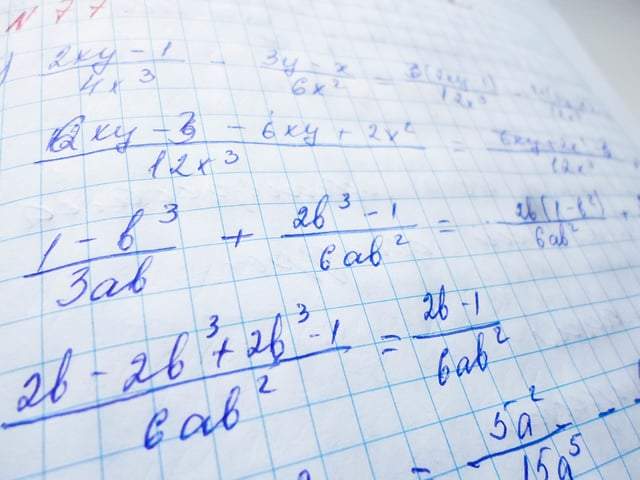# Formula Chart for the Passport to Advanced Math Questions on the SAT® Test

Just as there are requirements to get a passport to travel, you’ll need to fulfill a series of requirements if you want to ace the 16 Passport to Advanced Math Questions on the SAT® Test. The most important requirement is the ability to analyze, rewrite, and manipulate mathematical expressions, but that requires practice.

That’s why we have the tools you need at Union Test Prep! You can check our study guides and try your hand at our Passport to Advanced Math practice test. And you can check the following chart, which has the essential formulas you’ll need to manipulate and analyze and get your passport!

We also have formula charts for the other three areas of math covered on the SAT® Math Test:

But, for the Passport to Advanced Math questions, here are the necessary formulas:

Category Formula Symbols Comment
Passport to
Math
$$x+a=b \Rightarrow x=b-a$$
$$x-a=b \Rightarrow x=b+a$$
$$x \cdot a=b \Rightarrow x=b \div a$$
$$x \div a=b \Rightarrow x=b \cdot a$$
$$x^a=b \Rightarrow x = \sqrt[a]{b}$$
$$\sqrt[a]{x}= b \Rightarrow x= b^a$$
$$a^x=b \Rightarrow x=\frac{log\ b}{log\ a}$$
a, b = constants
x = variable

Passport to
Math
$$\dfrac{a}{b} + \dfrac{c}{d}= \dfrac{(a \cdot d)+(c \cdot b)}{(b \cdot d)}$$ a, b, c, d = any real number Remember to simplify
the fraction if possible.
Passport to
Math
$$\dfrac{a}{b} \cdot \dfrac{c}{d}= \dfrac{(a \cdot c)}{(b \cdot d)}$$ a, b, c, d = any real number Remember to simplify
the fraction if possible.
Passport to
Math
$$\dfrac{a}{b} \div \dfrac{c}{d}= \dfrac{(a \cdot d)}{(b \cdot c)}$$ a, b, c, d = any real number Remember to simplify
the fraction if possible.
Passport to
Math
$$a\dfrac{b}{c} = \dfrac{(a \cdot c)+b}{c}$$ a, b, c = any real number Remember to simplify
the fraction if possible.
Passport to
Math
$$x^a \cdot x^b = x^{a+b}$$ a, b, x = any real number
Passport to
Math
$$\dfrac{x^a}{x^b}=x^{a-b}$$ a, b, x = any real number
Passport to
Math
$$(x^a)^b=x^{a \cdot b}$$ a, x = any real number
Passport to
Math
$$(x \cdot y)^a=x^a \cdot y^a$$ a, b, x, y = any real number
Passport to
Math
$$x^1 = x$$ x = any real number
Passport to
Math
$$x^0 = 1$$ x = any real number
Passport to
Math
$$x^{-a} = \dfrac {1}{x^a}$$ a, x = any real number
Passport to
Math
$$x^{\frac {a}{b}} = \sqrt[b]{x^a} = (\sqrt[b]{x})^a$$ a, b, x = any real number
Passport to
Math
$$a^x =b \Rightarrow log_ab = x$$ a, b, x = any real number

Passport to
Math
$$ln(x) = log_ex$$ x = any real number
e = euler’s number

Passport to
Math
$$a^{log_ax} = x$$ a, x = any real number
Passport to
Math
$$log(a \cdot b) = log(a) + log(b)$$ a, b, = any real number
Passport to
Math
$$log(a \div b) = log(a) - log(b)$$ a, b, = any real number
Passport to
Math
$$log(a)^b = b \cdot log(a)$$ a, b, = any real number
Passport to
Math
$$log_ax= log_bx \cdot log_ab$$ a, b, x = any real number
Passport to
Math
$$log_ab = \dfrac{log_xb}{log_xa}$$ a, b, x = any real number
Passport to
Math
$$log_aa=1$$ a = any real number
Passport to
Math
$$log(1) = 0$$
Passport to
Math
$$(a \pm b)^2 =a^2 \pm 2 \cdot a \cdot b + b^2$$ a, b = constants or variables Square of sum or difference
Passport to
Math
$$a^2 - b^2 = (a+b)(a-b)$$ a, b = constants or variables Difference of
squares
Passport to
Math
$$a^3-b^3 = (a-b)(a^2 + ab + b^2)$$ a, b = constants or variables Difference of cubes
Passport to
Math
$$a^3+b^3 = (a+b)(a^2 - ab + b^2)$$ a, b = constants or variables Sum of cubes
Passport to
$$x = \dfrac{-b \pm \sqrt{b^2-4ac}}{2a}$$ a, b, c = constants
$$x = \dfrac{-b}{2a}$$ a, b = constants